# 股票数据爬虫进阶：免费、开源的股票爬虫Python库，实测真香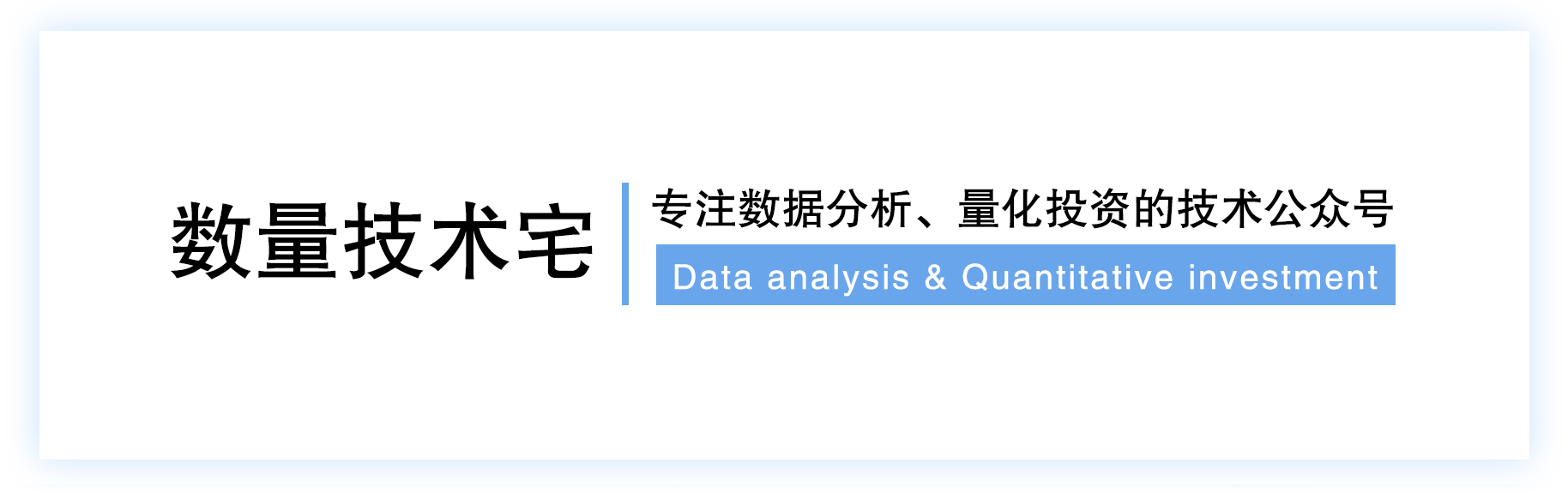## 免费、开源的股票爬虫Python库：Easyquotation

1. 新浪财经：全部A股实时行情（snapshot）

2. 集思录：分级基金、ETF、QDII实时数据

3. 腾讯财经：A股日内分时行情、港股日k线行情、港股实时行情（snapshot）

## 实测：新浪财经爬虫

import easyquotation
quotation = easyquotation.use('sina')
Res_Sina_AllSnap = quotation.market_snapshot(prefix=True)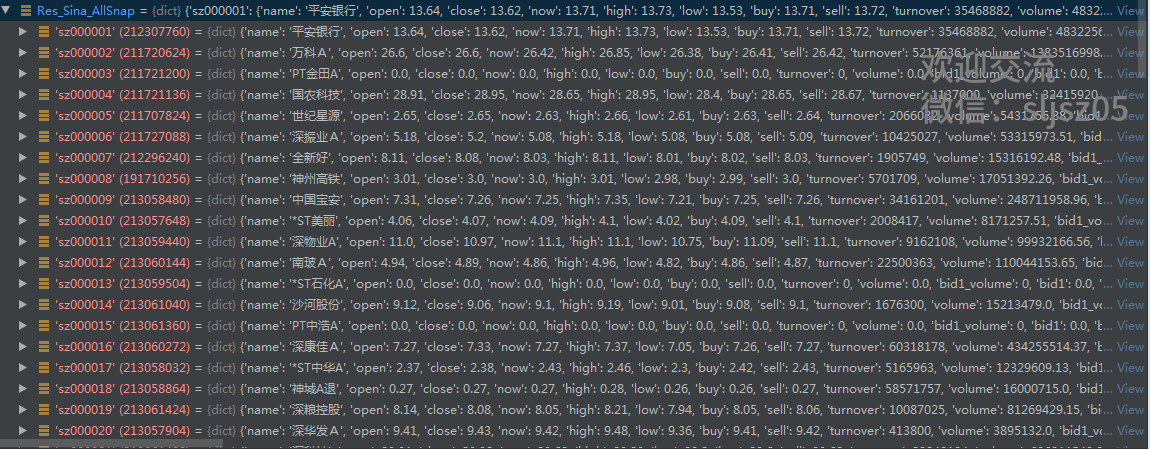Res_Sina_stock = quotation.real('513050')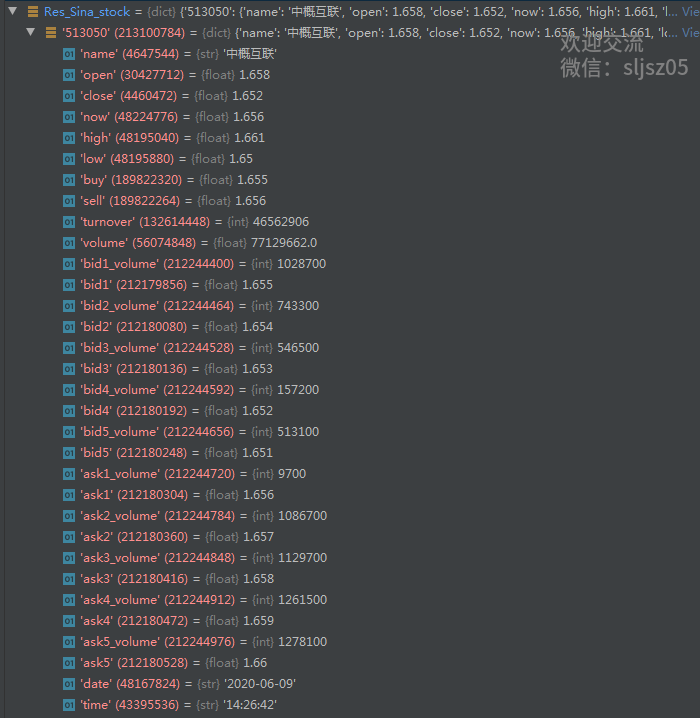Res_Sina_stocks = quotation.stocks(['600519', '601888'])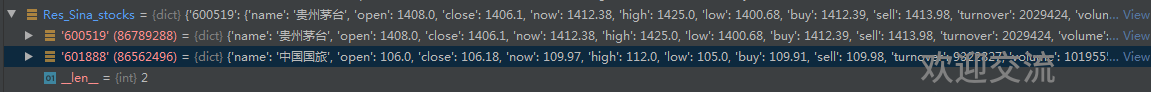Res_Sina_index = quotation.stocks(['sh000001', 'sz000001'], prefix=True)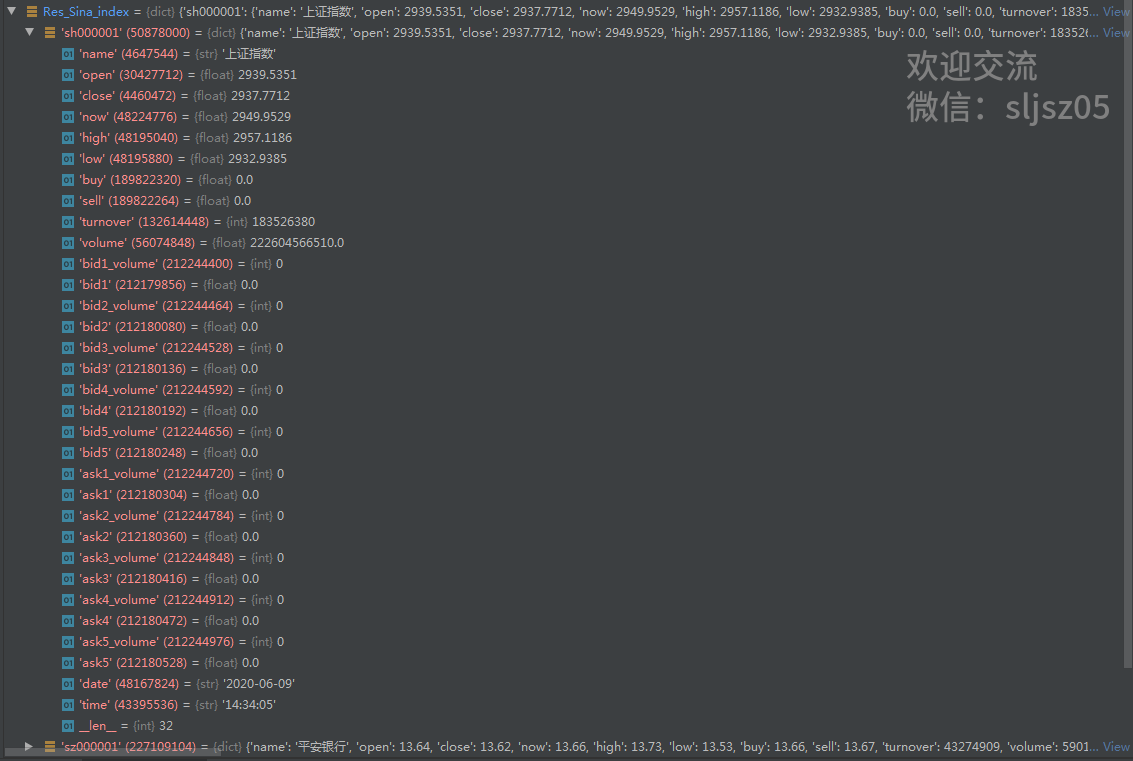## 实测：集思录爬虫

quotation = easyquotation.use('jsl')
Res_Jsl_fja = quotation.funda()
Res_Jsl_fjb = quotation.fundb()
Res_Jsl_qd = quotation.qdii()
Res_Jsl_etf = quotation.etfindex()
df_Jsl_fja = pd.DataFrame.from_dict(Res_Jsl_fja, orient='index')
df_Jsl_fjb = pd.DataFrame.from_dict(Res_Jsl_fjb, orient='index')
df_Jsl_qd = pd.DataFrame.from_dict(Res_Jsl_qd, orient='index')
df_Jsl_etf = pd.DataFrame.from_dict(Res_Jsl_etf, orient='index')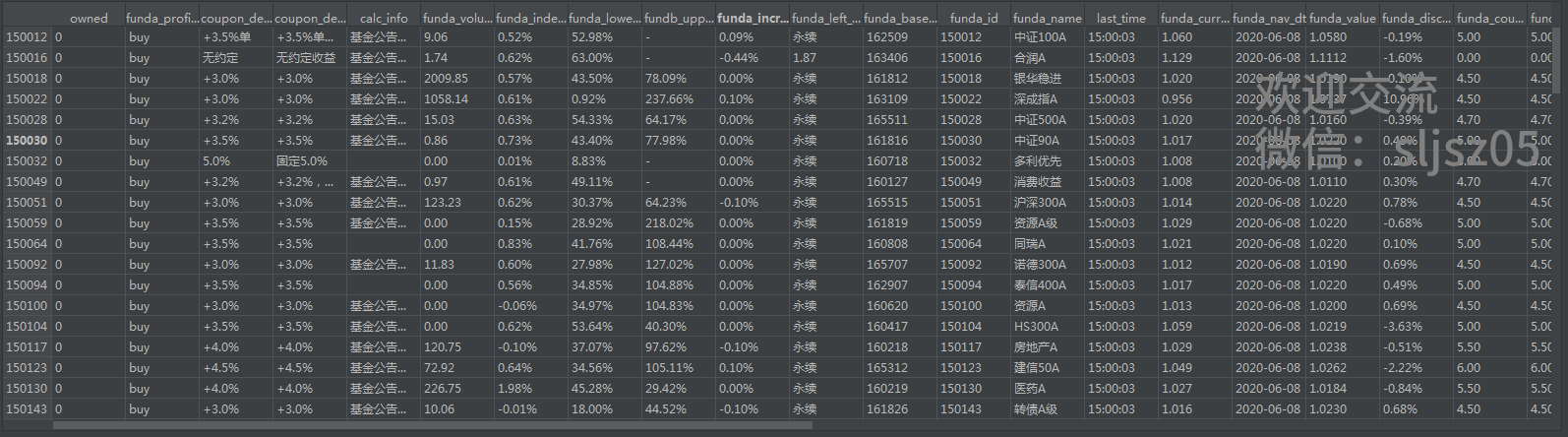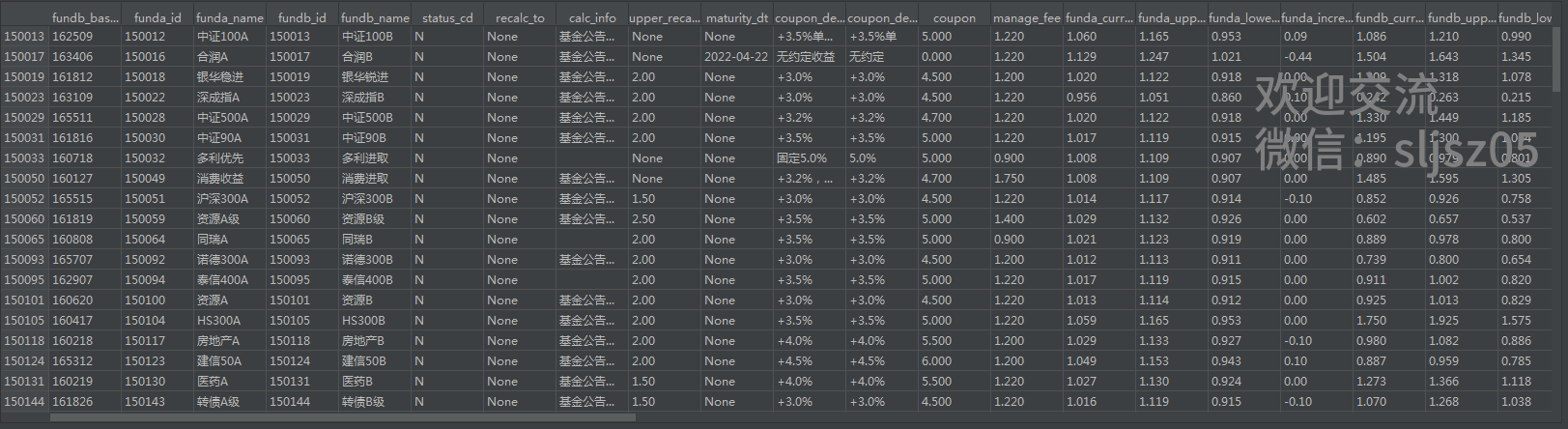ETF数据字段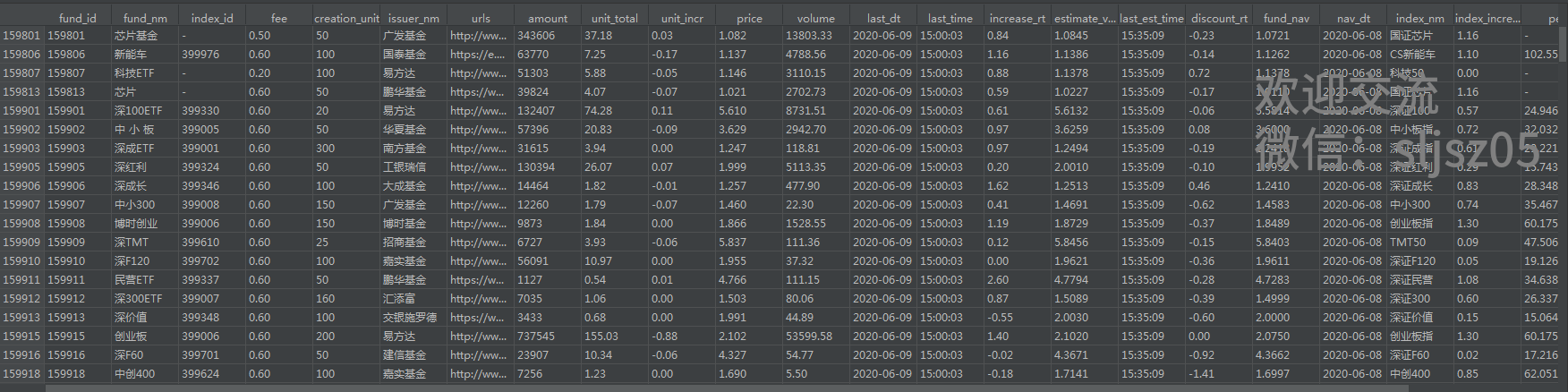QDII数据字段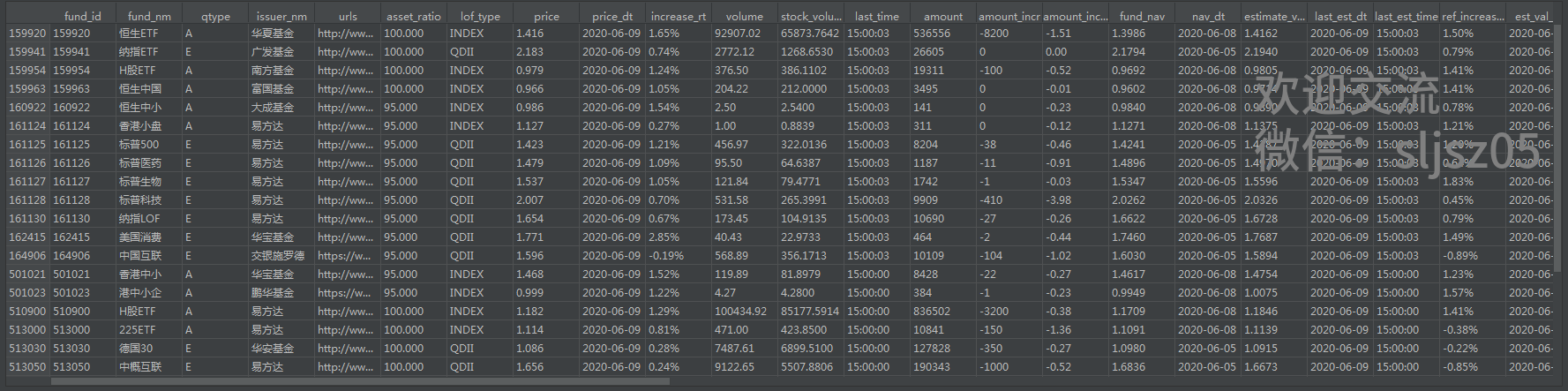## 实测：腾讯财经爬虫

quotation = easyquotation.use("timekline")
querycode = '600519'
Res_tx_mindata = quotation.real([querycode], prefix=True)
querykey = 'sh' + querycode + '.js'
mindata = pd.DataFrame(Res_tx_mindata[querykey]['time_data'], columns=['time', 'close', 'volume'])
mindata['close'] = mindata['close'].map(lambda x: float(x))
mindata['volume'] = mindata['volume'].map(lambda x: int(x))
fig, ax = plt.subplots(1, 1)
ax_sub = ax.twinx()  # 共享x轴，生成次坐标轴
l1, = ax.plot(mindata.time, mindata.close, 'r-', label='price')
l2, = ax_sub.plot(mindata.time, mindata.volume, 'b-', label='volume')
plt.legend(handles=[l1, l2], labels=['price', 'volume'], loc=0)
ax.set_ylabel('price')
ax_sub.set_ylabel('volume')
ax.set_xlabel('time')
ax.set_title('stock realtime')
x_major_locator = MultipleLocator(10)
ax.xaxis.set_major_locator(x_major_locator)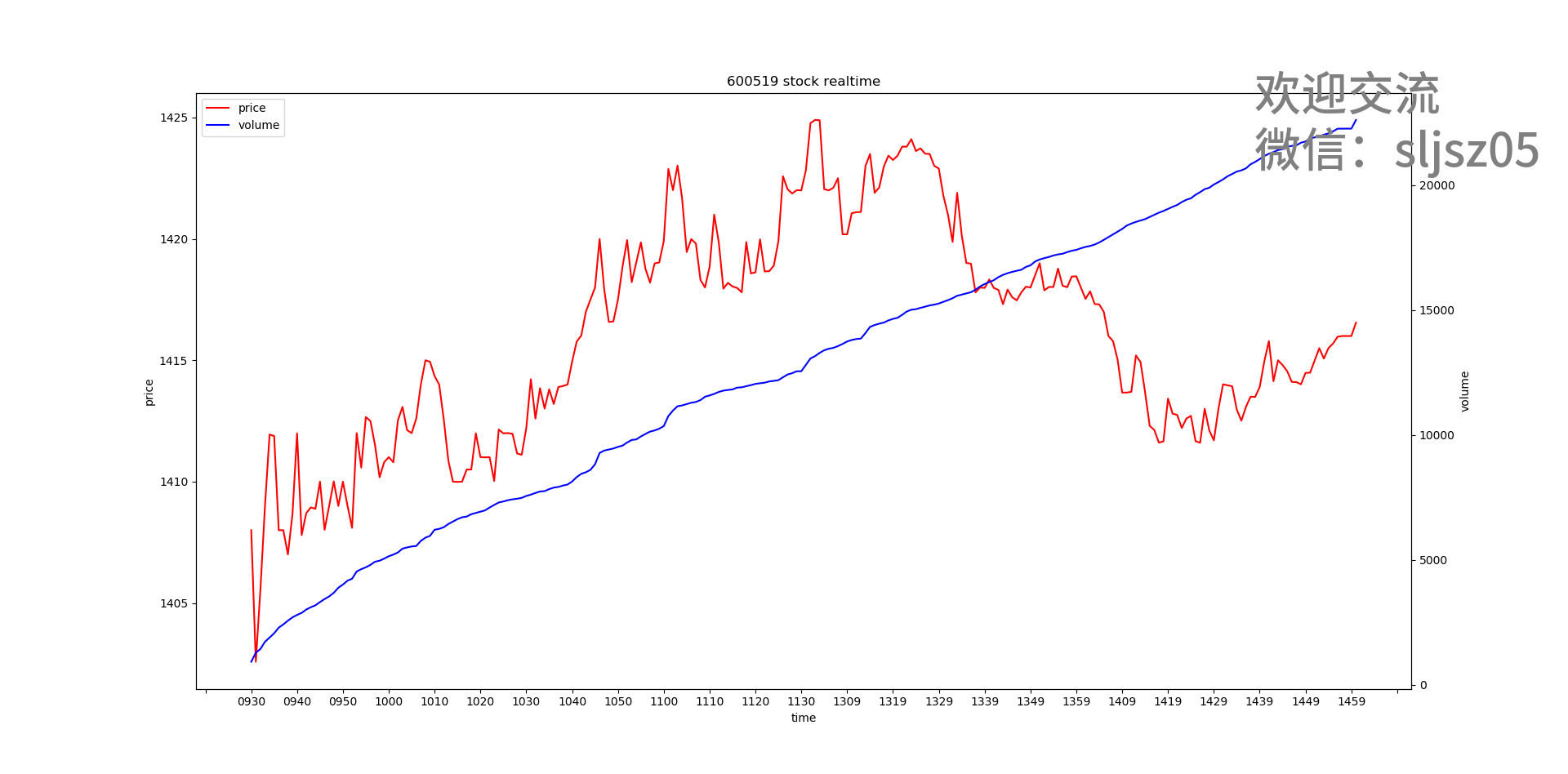querycode = '00700'
Res_tx_hk_dailydata = quotation.real([querycode])
hk_dailydata = pd.DataFrame(Res_tx_hk_dailydata[querycode],
columns=['Date', 'Open', 'Close', 'High', 'Low', 'volume', 'divi', 'none', 'amount'])
hk_dailydata['Close'] = hk_dailydata['Close'].map(lambda x: float(x))
hk_dailydata['volume'] = hk_dailydata['volume'].map(lambda x: float(x))
fig, ax = plt.subplots(1, 1)
ax_sub = ax.twinx()  # 共享x轴，生成次坐标轴
l1, = ax.plot(hk_dailydata.Date, hk_dailydata.Close, 'r-', label='price')
l2, = ax_sub.plot(hk_dailydata.Date, hk_dailydata.volume, 'b-', label='volume')
plt.legend(handles=[l1, l2], labels=['price', 'volume'], loc=0)
ax.set_ylabel('price')
ax_sub.set_ylabel('volume')
ax.set_xlabel('time')
ax.set_title(querycode + ' stock daily')
x_major_locator = MultipleLocator(120)
ax.xaxis.set_major_locator(x_major_locator)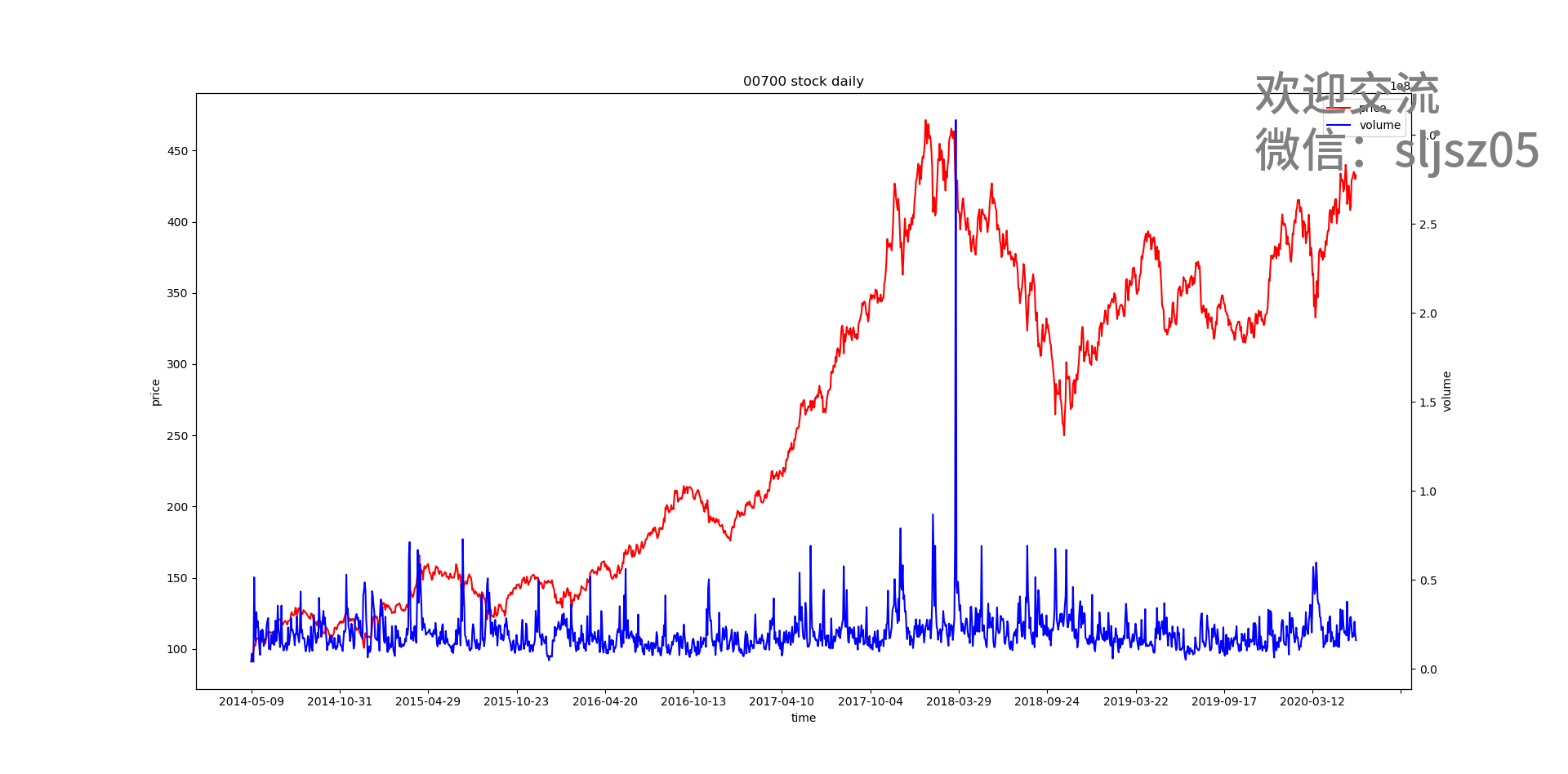quotation = easyquotation.use("hkquote")
Res_tx_hk_Snap = quotation.real(['00001', '00700'])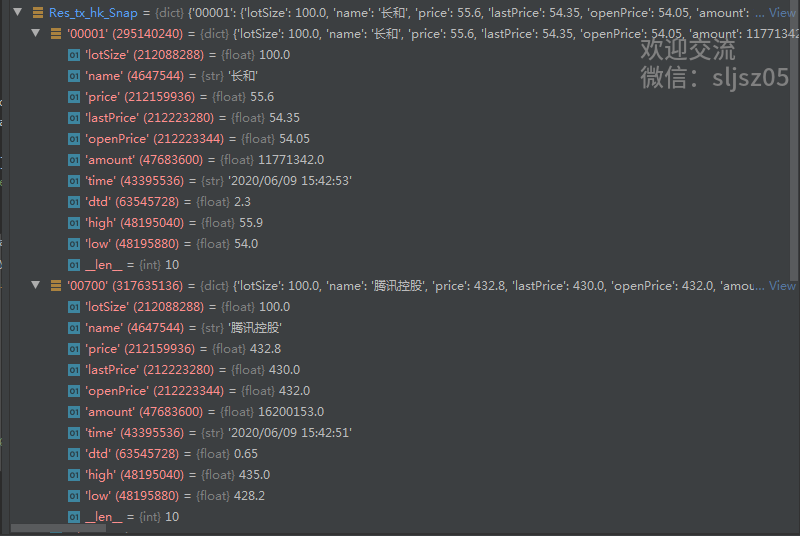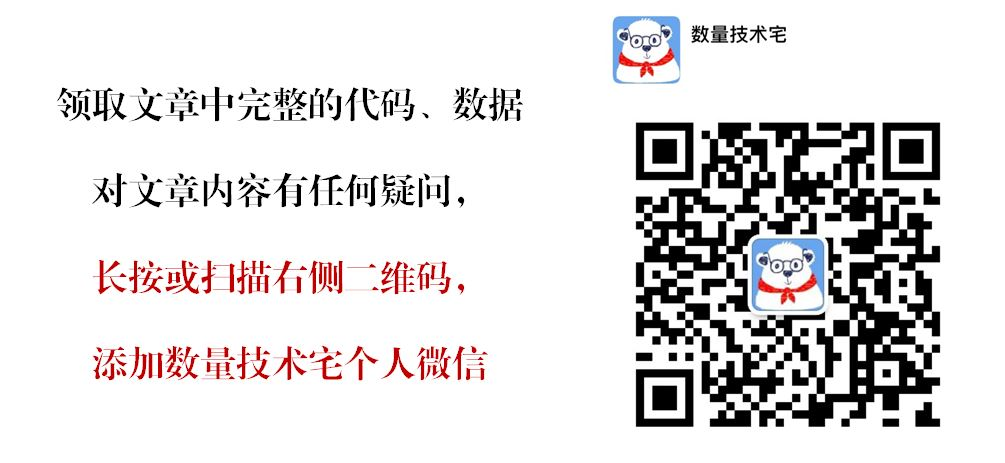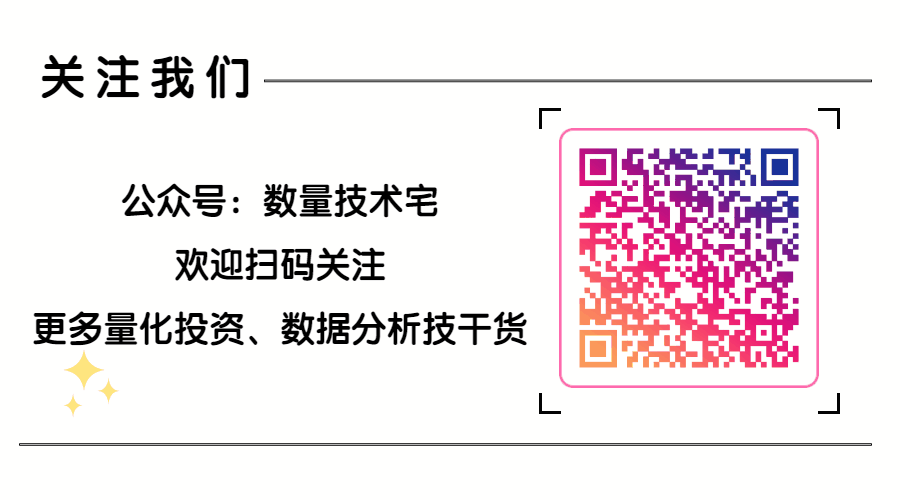## 往期干货分享推荐阅读

Omega System Trading and Development Club内部分享策略Easylanguage源码

AMA指标原作者Perry Kaufman 100+套交易策略源码分享

【数量技术宅|金融数据分析系列分享】为什么中证500（IC）是最适合长期做多的指数

【数量技术宅|金融数据分析系列分享】如何正确抄底商品期货、大宗商品

【数量技术宅|量化投资策略系列分享】股指期货IF分钟波动率统计策略

【数量技术宅 | Python爬虫系列分享】实时监控股市重大公告的Python爬虫

posted @ 2021-11-20 18:45  数量技术宅  阅读(1543)  评论(0编辑  收藏  举报